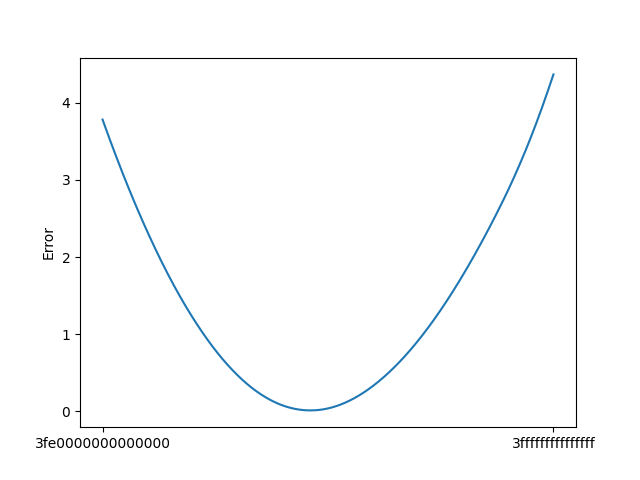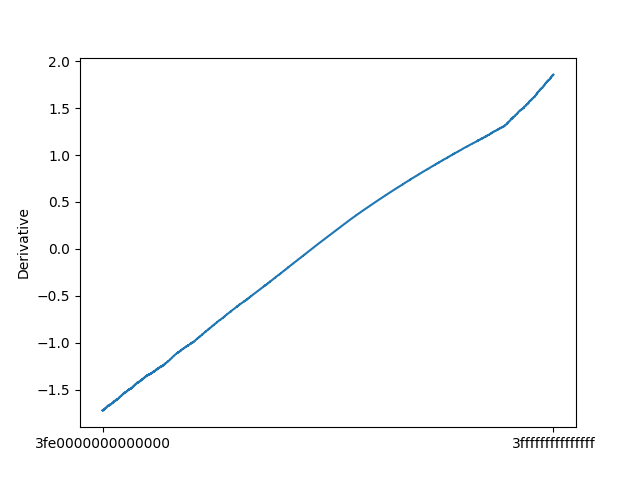# 快速平方根算法 - 从卡马克的魔法数字谈起

### 牛顿迭代

• 首先选择一个接近方程根的点 $x_0$，计算得到 $f(x_0)$ 与 $f’(x_0)$

• 计算函数在该点处的切线与 $x$ 轴的交点：
$$(x - x_0) \cdot f’(x_0) + f(x_0) = 0$$

• 解出 $x$，并将其记为 $x_1$，这个点通常会比 $x_0$ 更接近方程根，于是可以不断的迭代上面的方法进行逼近

• 对于给定的精度 $\epsilon$，重复上面的方法，直到：
$$x_n - x_{n - 1} \leq \epsilon$$

$$f(x) = \sum_{n = 0}^{\infty} \frac{ f^{(n)}(x_0) }{n!} (x - x_0)^n = f(x_0) + f’(x_0)(x - x_0) + \dots$$### 初步实现

$$f’(x_n) = 2x_n \Rightarrow x_{n + 1} = x_n - \frac{f(x_n)}{f’(x_n)} = x_n - \frac{x_n}{2} + \frac{a}{2x_n} = \frac{1}{2} \left( x_n + \frac{a}{x_n} \right)$$

### 浮点数的内存布局$$3.125_\text{d} = 2^{0} + 2^{1} + 2^{-3} = 11.001_\text{b} = 1.1001 ^ {1}_{b}$$

$$0_{\text{sign}} \ 10000000000_{\text{exp}} \ 100100000000000000000000000000000000000000000000000_{\text{frac}}$$

### 第一个魔法数字

double 取地址转为 64 位整形指针，除以 2 则用位移实现：

### 魔法数字的状态空间

$$(\text{0x3fe0000000000000}, \text{0x3fffffffffffffff})$$

• 首先将牛顿迭代的次数改为一次，这样针对性的优化版本可以得到常数的时间复杂度
• 在函数中动态的使用可以更改的魔法数字
• 将其编译为可供 ctypes 使用的函数，并使用 numpy 进行包装

• 对指定的魔法数字，在一段空间内随机抽取一部分数字
• 使用每一个随机抽取的数字，计算一次牛顿牛顿迭代后的值与真实根值的差
• 对差求得平均值作为该魔法数字的误差值### 梯度下降

• 对于每一个给定的魔法数字，按照上面的方法求得左右侧一段范围的一个数对应的误差
• 如果该数字单侧的距离小于这个范围，那么只使用单侧的误差值
• 之后使用（右侧误差值 - 左侧误差值）/（2 * 区间长度）定义为该值的导数值
• 只要区间相对于整体很小，那么这个导数值还是较为准确的### 参考资料

Double-precision floating-point format - Wikipedia

Newton’s method - Wikipedia